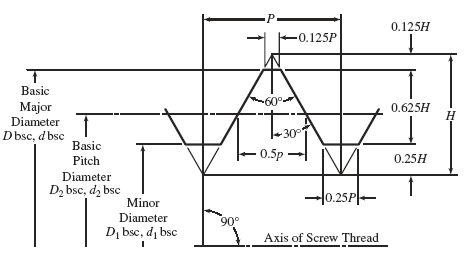Related Resources: calculators

### Screw Threads Calculator for Internal Unified Inch Screw

UN Internal Screw Threads Formulas and Calculator per. ASME B1.1

The formulas below are for inch screw threads are based on those listed in ASME B1.1, Unified Inch Screw Threads.Formulas Used:
Note that calculated values may be slightly different than table reference data due to math rounding differences.

Minimum internal minor diameter

Eq. 1
D1min. = Dbsc - 2hn

Eq. 2
2hn = 1.08253175P

where

Dbsc = Basic major diameter
2hn = double height of internal thread
P =  1/n

Eq. 3
D1max. = D1min. + TD1

Eq. 4
TD1 = 0.25P - 0.4P2

where

D1 max. = Maximum internal minor diameter
D1 min. = Minimum internal minor diameter
TD1 = internal minor diameter tolerance

Eq. 5
D2 min. = Dbsc − hb

Eq. 6
hb = 0.64951905P

where

D2min. = Minimum internal pitch diameter
D bsc = Basic major diameter

Eq. 7
D2max. = D2 min. + TD2

Eq 8.
TD2 = 1 .300 Td2

Eq. 9
Td2 = 0.0015 (d bsc)1/3 + 0.0015 LE1/2 0.015 (P2)1/3

where

D2 max. = Maximum internal pitch diameter
D2 min. = Minimum internal pitch diameter
TD2 = internal pitch diameter tolerance
LE = length of engagement

Eq 10
D min. = D bsc

where

Dmin. = Minimum internal major diameter
Dbsc = Basic major diameter

Notes

(a) Maximum Major Diameter (Internal Threads ). In dimensioning internal threads, the maximum major diameter is not specified, being established by the crest of an unworn tool. In practice, the major diameter of an internal thread is satisfactory when accepted by a gage or gaging method that represents the maximum material condition of an external thread that has no allowance.

(b) Minimum Major Diameter (Internal Threads), Dbsc. For all classes, equals basic diameter Dbsc.

(c) Minimum Pitch Diameter. For all classes, D2bsc equals basic pitch diameter D2 minimum.

(d) Maximum Pitch Diameter (Internal Threads). For all classes, equals minimum pitch diameter D2bsc, plus pitch diameter tolerance for respective class of thread

(e) Minimum Minor Diameter (Internal Threads). For all classes, D1bsc equals minimum pitch diameter D2 minimum minus 0.43301122P, then rounded off to the nearest 0.001 in. for sizes 0.138 in. and larger. For Class 3B, a cipher is added to yield four decimal places.

(f) Maximum Minor Diameter (Internal Threads) . All classes are calculated before rounding, then rounded for Classes 1B and 2B to the nearest 0.001 in. for sizes 0.138 in. and larger. Class 3B values are rounded to four decimal places.

Plating considerations

Standard internal threads provide no allowance for coating thickness. To determine before coating gaging limits for a coated thread, increase

(a) the minimum pitch diameter by 4 times the maximum coating thickness, if specified, or by 6 times the minimum or nominal coating thickness, if not specified.

(b) the maximum pitch diameter by 4 times the minimum or nominal coating thickness.

(c) the minimum minor diameter by 2 times the maximum coating thickness, if specified, or by 3 times the minimum or nominal coating thickness, if not specified.

(d) the maximum minor diameter by 2 times the minimum or nominal coating thickness.

Related: